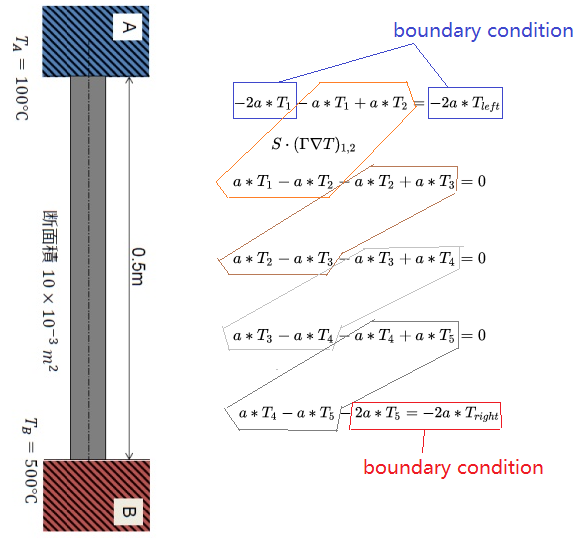OpenFOAM中的数学模型，最终是要解线性方程组Ax=b

fvScalarMatrix TEqn(fvm::laplacian(k, T));
TEqn.solve();


// Assigning diagonal coefficients
for(label i=0; i<Nc; i++)
{
A[i][i] = TEqn.diag()[i];
}
// Assigning off-diagonal coefficients
{
A[l][u] = TEqn.upper()[faceI];
A[u][l] = TEqn.upper()[faceI];
}
// Assigning contribution from BC
forAll(T.boundaryField(), patchI)
{
const fvPatch &pp = T.boundaryField()[patchI].patch();
forAll(pp, faceI)
{
label cellI = pp.faceCells()[faceI];
A[cellI][cellI] += TEqn.internalCoeffs()[patchI][faceI];
A.source()[cellI] += TEqn.boundaryCoeffs()[patchI][faceI];
}
}


A[l][u] = TEqn.upper()[faceI];
A[u][l] = TEqn.lower()[faceI];


source项没有用上应该是因为这个公式里没有source，所以可以省略，真实计算时应该有：

A.source()=TEqn.source()

fvScalarMatrix TEqn(fvm::laplacian(k, T));
Info<< "TEqn = " << TEqn << endl;
TEqn.solve();
Info<< "T = " << T << endl;


//输出lower，diag和upper
TEqn = false true true 5(-100 -200 -200 -200 -100)4(100 100 100 100)
//输出dimension，不重要
[1 2 -3 0 0 0 0]
//输出source，也就是b
5{0}
//输出internalCoeffs
4
(
1(-200)
1(-200)
0()
0()
)

//输出boundaryCoeffs
4
(
1(-20000)
1(-100000)
0()
0()
)

DICPCG:  Solving for T, Initial residual = 1, Final residual = 1.21266e-16, No Iterations 1
//输出更新后的T
T = dimensions      [0 0 0 1 0 0 0];

internalField   nonuniform List<scalar> 5(140 220 300 380 460);

boundaryField
{
left
{
type            fixedValue;
value           uniform 100;
}
right
{
type            fixedValue;
value           uniform 500;
}
topAndBottom
{
type            empty;
}
frontAndBack
{
type            empty;
}
}


¥n==Coefficient Matrix==
-300 100 0 0 0 -20000
100 -200 100 0 0 0
0 100 -200 100 0 0
0 0 100 -200 100 0
0 0 0 100 -300 -100000
Solution: 5(140 220 300 380 460)

• 显示离散化矩阵的元素值；
• 显示cell和boundary上的值。

Contrib gdbOF - OpenFOAMWikiopenfoamwiki.net

cd \$WM_PROJECT_DIR
wclean all
./Allwmake

cd mylaplacianFoam
wclean && wmake

exec-file mylaplacianFoam

pfvmatrixfull this matrix.txt 1 1 3 3

TEqn(fvm::laplacian(k,T));

• gaussLaplacianSchemes.C (算b)
• gaussLaplacianScheme.C (算A)

gaussLaplacianSchemes.C里把干活的函数定义成macro(宏)了，这非常讨厌，因为写成宏的function就不能用gdb一步一步测试了。

gdb mylaplacianFoam
b gaussLaplacianScheme.C:52
r

deltaCoeffs在宏里面，我看不到。我估计就是1/d，d是两个cell中心的间距。

Therefore, the coefficients calculated in the following line are gamma_f * |Delta|/|d|, where Delta is the vector defined in the Dr. Jasak's thesis(Eq. (3.32)).
fvm.upper() = deltaCoeffs.internalField()*gammaMagSf.internalField();fvm.lower is never mentioned - this is because it is equal to fvm.upper and a symmetric matrix is being created.

• 假如两个都没被赋值，被呼叫时就会赋值0；
• 假如其中一个被呼叫时自己有值，则不做动作。
Foam::scalarField& Foam::lduMatrix::lower()
{
if (!lowerPtr_)
{
if (upperPtr_)
{
lowerPtr_ = new scalarField(*upperPtr_);
}
else
{
}
}

return *lowerPtr_;
}///////////////////////////////////////////////////////////////////////////Jasak的论文里介绍了3种非正交修正的方法，OpenFOAM里用的是第三种。区别就是delta的定义不同。

-----------------------------------------------------------------

（Jasak，OpenFOAM的主要作者之一(35%)，克罗地亚人，1993年在伦敦帝国学院(也叫做帝国理工)读PhD期间开始参与写FOAM。后来回克罗地亚当了教授，又由于理念不合跟另一个主要作者Henry(60%)闹翻了，自己搞了一个Wikki公司。他的博士论文是OpenFOAM的入门经典教程之一。）

http://powerlab.fsb.hr/ped/kturbo/OpenFOAM/docs/HrvojeJasakPhD.pdfpowerlab.fsb.hr

https://www.cfd-online.com/Forums/openfoam/152605-original-openfoam-author-s.htmlwww.cfd-online.com

-----------------------------------------------------------------

laplacianSchemes里定义的那个corrected就是用来控制算不算非正交修正的。corrected就表示要算，uncorrected就表示不要算。